# R tutorials for Business Analyst – Bar Chart and Histogram in R

A bar chart is a great way to display categorical variables in the x-axis. This type of graph denotes two aspects in the y-axis.

1. The first one counts the number of occurrence between groups.
2. The second one shows a summary statistic (min, max, average, and so on) of a variable in the y-axis.

You will use the mtcars dataset with has the following variables:

• cyl: Number of the cylinder in the car. Numeric variable
• am: Type of transmission. 0 for automatic and 1 for manual. Numeric variable
• mpg: Miles per gallon. Numeric variable

In this tutorial, you will learn

• How to create Bar Chart
• Change the color of the bars
• Change the intensity
• Color by groups
• Add a group in the bars
• Bar chart in percentage
• Side by side bars
• Histogram

## How to create Bar Chart

To create graph in R, you can use the library ggplot which creates ready-for-publication graphs. The basic syntax of this library is:

```ggplot(data, mapping = aes()) +
geometric object

arguments:
data: dataset used to plot the graph
mapping: Control the x and y-axis
geometric object: The type of plot you want to show. The most common objects are:

- Point: `geom_point()`
- Bar: `geom_bar()`
- Line: `geom_line()`
- Histogram: `geom_histogram()`
```

In this tutorial, you are interested in the geometric object geom_bar() that create the bar chart.

## Bar chart: count

Your first graph shows the frequency of cylinder with geom_bar(). The code below is the most basic syntax.

```library(ggplot2)
# Most basic bar chart
ggplot(mtcars, aes(x = factor(cyl))) +
geom_bar()
```

Code Explanation

• You pass the dataset mtcars to ggplot.
• Inside the aes() argument, you add the x-axis as a factor variable(cyl)
• The + sign means you want R to keep reading the code. It makes the code more readable by breaking it.
• Use geom_bar() for the geometric object.

Output:Note: make sure you convert the variables into a factor otherwise R treats the variables as numeric. See the example below.## Customize the graph

Four arguments can be passed to customize the graph:

```- `stat`: Control the type of formatting. By default, `bin` to plot a count in the y-axis. For continuous value, pass `stat = "identity"`
- `alpha`: Control density of the color
- `fill`: Change the color of the bar
- `size`: Control the size the bar
```

### Change the color of the bars

You can change the color of the bars. Note that the colors of the bars are all similar.

```# Change the color of the bars
ggplot(mtcars, aes(x = factor(cyl))) +
geom_bar(fill = "coral") +
theme_classic()
```

Code Explanation

• The colors of the bars are controlled by the aes() mapping inside the geometric object (i.e. not in the ggplot()). You can change the color with the fill arguments. Here, you choose the coral color.

Output:You can use this code:

`grDevices::colors()`

to see all the colors available in R. There are around 650 colors.

### Change the intensity

You can increase or decrease the intensity of the bars’ color

```# Change intensity
ggplot(mtcars,
aes(factor(cyl))) +
geom_bar(fill = "coral",
alpha = 0.5) +
theme_classic()
```

Code Explanation

• To increase/decrease the intensity of the bar, you can change the value of the alpha. A large alpha increases the intensity, and low alpha reduces the intensity. alpha ranges from 0 to 1. If 1, then the color is the same as the palette. If 0, color is white. You choose alpha = 0.1.

Output:### Color by groups

You can change the colors of the bars, meaning one different color for each group. For instance, cyl variable has three levels, then you can plot the bar chart with three colors.

```# Color by group
ggplot(mtcars, aes(factor(cyl),
fill = factor(cyl))) +
geom_bar()
```

Code Explanation

• The argument fill inside the aes() allows changing the color of the bar. You change the color by setting fill = x-axis variable. In your example, the x-axis variable is cyl; fill = factor(cyl)

Output:### Add a group in the bars

You can further split the y-axis based on another factor level. For instance, you can count the number of automatic and manual transmission based on the cylinder type.

You will proceed as follow:

• Step 1: Create the data frame with mtcars dataset
• Step 2: Label the am variable with auto for automatic transmission and man for manual transmission. Convert am and cyl as a factor so that you don’t need to use factor() in the ggplot() function.
• Step 3: Plot the bar chart to count the number of transmission by cylinder

```library(dplyr)
# Step 1
data <- mtcars % > %
#Step 2
mutate(am = factor(am, labels = c("auto", "man")),
cyl = factor(cyl))
```

You have the dataset ready, you can plot the graph;

# Step 3

```ggplot(data, aes(x = cyl, fill = am)) +
geom_bar() +
theme_classic()
```

Code Explanation

• The ggpplot() contains the dataset data and the aes().
• In the aes() you include the variable x-axis and which variable is required to fill the bar (i.e. am)
• geom_bar(): Create the bar chart

Output: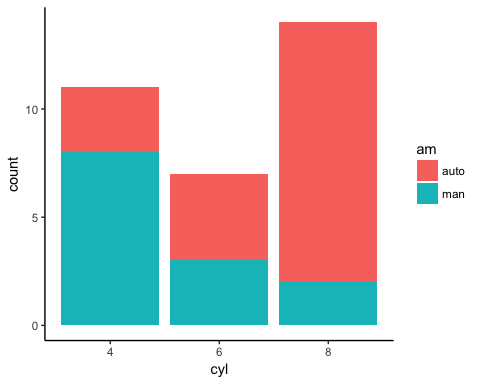The mapping will fill the bar with two colors, one for each level. It is effortless to change the group by choosing other factor variables in the dataset.

### Bar chart in percentage

You can visualize the bar in percentage instead of the raw count.

# Bar chart in percentage

```ggplot(data, aes(x = cyl, fill = am)) +
geom_bar(position = "fill") +
theme_classic()
```

Code Explanation

• Use position = “fill” in the geom_bar() argument to create a graphic with percentage in the y-axis.

Output: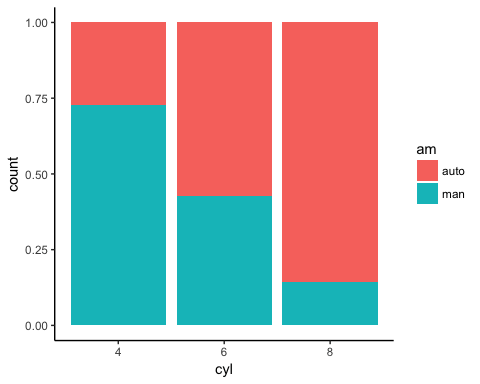### Side by side bars

It is easy to plot the bar chart with the group variable side by side.

```# Bar chart side by side
ggplot(data, aes(x = cyl, fill = am)) +
geom_bar(position = position_dodge()) +
theme_classic()
```

Code Explanation

• position=position_dodge(): Explicitly tells how to arrange the bars

Output: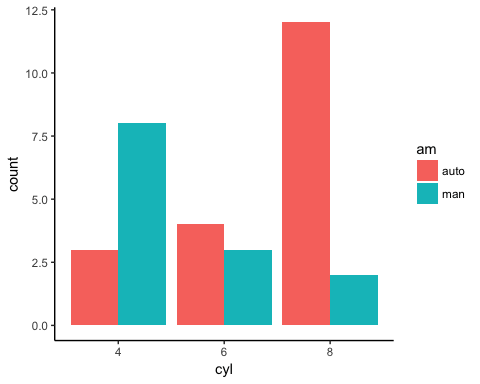## Histogram

In the second part of the bar chart tutorial, you can represent the group of variables with values in the y-axis.

Your objective is to create a graph with the average mile per gallon for each type of cylinder. To draw an informative graph, you will follow these steps:

• Step 1: Create a new variable with the average mile per gallon by cylinder
• Step 2: Create a basic histogram
• Step 3: Change the orientation
• Step 4: Change the color
• Step 5: Change the size
• Step 6: Add labels to the graph

Step 1) Create a new variable

You create a data frame named data_histogram which simply returns the average miles per gallon by the number of cylinders in the car. You call this new variable mean_mpg, and you round the mean with two decimals.

# Step 1

```data_histogram <- mtcars % > %
mutate(cyl = factor(cyl)) % > %
group_by(cyl) % > %
summarize(mean_mpg = round(mean(mpg), 2))
```

Step 2) Create a basic histogram

You can plot the histogram. It is not ready to communicate to be delivered to client but gives us an intuition about the trend.

```ggplot(data_histogram, aes(x = cyl, y = mean_mpg)) +
geom_bar(stat = "identity")
```

Code Explanation

• The aes() has now two variables. The cyl variable refers to the x-axis, and the mean_mpg is the y-axis.
• You need to pass the argument stat=”identity” to refer the variable in the y-axis as a numerical value. geom_bar uses stat=”bin” as default value.

Output: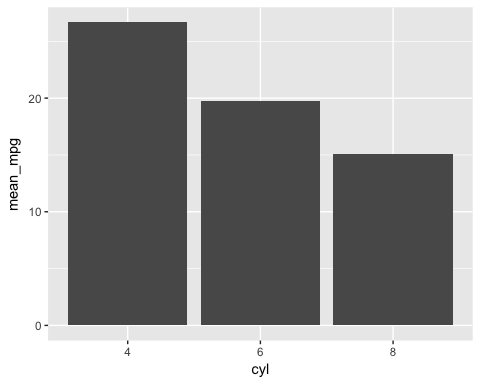Step 3) Change the orientation

You change the orientation of the graph from vertical to horizontal.

```ggplot(data_histogram, aes(x = cyl, y = mean_mpg)) +
geom_bar(stat = "identity") +
coord_flip()
```

Code Explanation

• You can control the orientation of the graph with coord_flip().

Output:Step 4) Change the color

You can differentiate the colors of the bars according to the factor level of the x-axis variable.

```ggplot(data_histogram, aes(x = cyl, y = mean_mpg, fill = cyl)) +
geom_bar(stat = "identity") +
coord_flip() +
theme_classic()
```

Code Explanation

• You can plot the graph by groups with the fill= cyl mapping. R takes care automatically of the colors based on the levels of cyl variable

Output: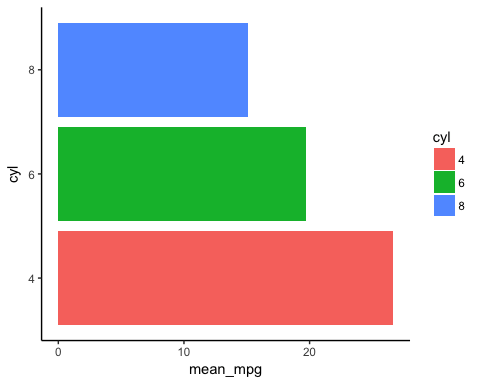Step 5) Change the size

To make the graph looks prettier, you reduce the width of the bar.

```graph <- ggplot(data_histogram, aes(x = cyl, y = mean_mpg, fill = cyl)) +
geom_bar(stat = "identity",
width = 0.5) +
coord_flip() +
theme_classic()
```

Code Explanation

• The width argument inside the geom_bar() controls the size of the bar. Larger value increases the width.
• Note, you store the graph in the variable graph. You do so because the next step will not change the code of the variable graph. It improves the readability of the code.

Output: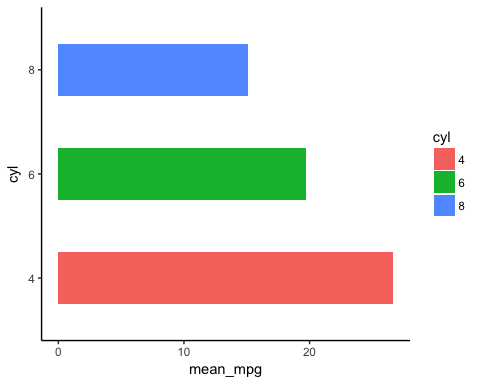Step 6) Add labels to the graph

The last step consists to add the value of the variable mean_mpg in the label.

```graph +
geom_text(aes(label = mean_mpg),
hjust = 1.5,
color = "white",
size = 3) +
theme_classic()
```

Code Explanation

• The function geom_text() is useful to control the aesthetic of the text.
• label=: Add a label inside the bars
• mean_mpg: Use the variable mean_mpg for the label
• hjust controls the location of the label. Values closed to 1 displays the label at the top of the bar, and higher values bring the label to the bottom. If the orientation of the graph is vertical, change hjust to vjust.
• color=”white”: Change the color of the text. Here you use the white color.
• size=3: Set the size of the text.

Output:## Summary

A bar chart is useful when the x-axis is a categorical variable. The y-axis can be either a count or a summary statistic. The table below summarizes how to control bar chart with ggplot2:

Objective code
Count
`ggplot(df, eas(x= factor(x1)) + geom_bar()`
Count with different color of fill
`ggplot(df, eas(x= factor(x1), fill = factor(x1))) + geom_bar()`
Count with groups, stacked
`ggplot(df, eas(x= factor(x1), fill = factor(x2))) + geom_bar(position=position_dodge())`
Count with groups, side by side
`ggplot(df, eas(x= factor(x1), fill = factor(x2))) + geom_bar()`
Count with groups, stacked in %
`ggplot(df, eas(x= factor(x1), fill = factor(x2))) + geom_bar(position=position_dodge())`
Values
`ggplot(df, eas(x= factor(x1)+ y = x2) + geom_bar(stat="identity")`

# Personal Career & Learning Guide for Data Analyst, Data Engineer and Data Scientist

## Applied Machine Learning & Data Science Projects and Coding Recipes for Beginners

A list of FREE programming examples together with eTutorials & eBooks @ SETScholars

# Projects and Coding Recipes, eTutorials and eBooks: The best All-in-One resources for Data Analyst, Data Scientist, Machine Learning Engineer and Software Developer

Topics included: Classification, Clustering, Regression, Forecasting, Algorithms, Data Structures, Data Analytics & Data Science, Deep Learning, Machine Learning, Programming Languages and Software Tools & Packages.
(Discount is valid for limited time only)`Disclaimer: The information and code presented within this recipe/tutorial is only for educational and coaching purposes for beginners and developers. Anyone can practice and apply the recipe/tutorial presented here, but the reader is taking full responsibility for his/her actions. The author (content curator) of this recipe (code / program) has made every effort to ensure the accuracy of the information was correct at time of publication. The author (content curator) does not assume and hereby disclaims any liability to any party for any loss, damage, or disruption caused by errors or omissions, whether such errors or omissions result from accident, negligence, or any other cause. The information presented here could also be found in public knowledge domains.`

# Learn by Coding: v-Tutorials on Applied Machine Learning and Data Science for Beginners

Please do not waste your valuable time by watching videos, rather use end-to-end (Python and R) recipes from Professional Data Scientists to practice coding, and land the most demandable jobs in the fields of Predictive analytics & AI (Machine Learning and Data Science).

The objective is to guide the developers & analysts to “Learn how to Code” for Applied AI using end-to-end coding solutions, and unlock the world of opportunities!

BAR plot in R | Jupyter Notebook | R Data Science for beginners

How to create bar chart in R

Python Data Visualisation for Business Analyst – How to do Density plot in Python